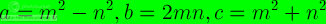# NumPy Project Euler Problem 9

This entry is part 9 of 9 in the series NumPy Project Euler

Project Euler Problem 9 is a tough one. After reading the Pythagorean Triple Wikipedia page, I implemented a NumPy solution with Euclid’s Formula.### 1. Create m and n arrays

The Euclid’s Formula defines indices m and n. We will create arrays to hold these indices.

```1 2 m = numpy.arange(33) n = numpy.arange(33)```

### 2. Calculate a, b and c

The second step is to calculate a, b and c of the Pythagorean triple using Euclid’s formula. Use the outer function to get the cartesian products, difference and sums we require.

```1 2 3 a = numpy.subtract.outer(m ** 2, n ** 2) b = 2 * numpy.multiply.outer(m, n) c = numpy.add.outer(m ** 2, n ** 2)```

### 3. Find the index

At this point we have a number of arrays containing a, b and c values. However, we still need to find the values that conform to the problem’s condition. Find the index of those values with the NumPy where function.

```1 idx = numpy.where((a + b + c) == 1000)```

### 4. Check solution

Check the solution with the numpy.testing module.

```1 numpy.testing.assert_equal(a[idx]**2 + b[idx]**2, c[idx]**2)```

Below is the complete code or get it from Github

```1 2 3 4 5 6 7 8 9 10 11 12 13 14 15 16 17 18 19 20 21 22 23 24 25 26 import numpy import numpy.testing   #A Pythagorean triplet is a set of three natural numbers, a < b < c, for which, #a ** 2 + b ** 2 = c ** 2 # #For example, 32 + 42 = 9 + 16 = 25 = 52. # #There exists exactly one Pythagorean triplet for which a + b + c = 1000. #Find the product abc.   #1. Create m and n arrays m = numpy.arange(33) n = numpy.arange(33)   #2. Calculate a, b and c a = numpy.subtract.outer(m ** 2, n ** 2) b = 2 * numpy.multiply.outer(m, n) c = numpy.add.outer(m ** 2, n ** 2)   #3. Find the index idx = numpy.where((a + b + c) == 1000)   #4. Check solution numpy.testing.assert_equal(a[idx]**2 + b[idx]**2, c[idx]**2) print a[idx] * b[idx] * c[idx]```

If you liked this post and are interested in NumPy check out NumPy Beginner’s Guide by yours truly.

Series NavigationNumPy Project Euler Problem 8
By the author of NumPy Beginner's Guide, NumPy Cookbook and Instant Pygame. If you enjoyed this post, please consider leaving a comment or subscribing to the RSS feed to have future articles delivered to your feed reader.
This entry was posted in programming and tagged , , , . Bookmark the permalink.